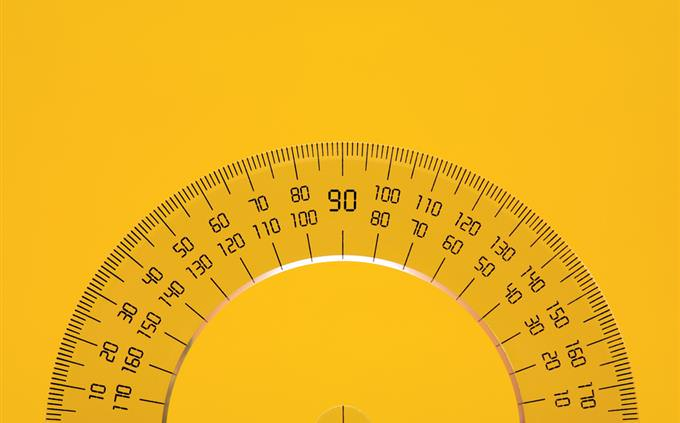# Do You Remember Your Basic Geometry?

Geometry is the step sister of the regular math we do every day. True, most of us make very little use of geometry in the usual course of our lives, but those lessons stuck with me, and I found that quite a few questions rang some very old bells. So how about it? Do you think you remember enough to beat our quiz?A triangle has one angle that is 60 degrees, what is the sum of the degrees of the other two angles?
120
180
160
No way of knowingA reflex angle is an angle that is...
More than 180 but less than 360 degrees
More than 90 but less than 180 degrees
More than 270 but less than 360 degrees
More than 60 but less than 180 degreesHow does one measure the AREA of a triangle?
Base multiplied by height divided by 2
Height multiplied by sum of angles divided by 3
Base multiplied by sum of angled divided by 3
Height multiplied by largest angle, divided by 2How does one calculate the area of a rectangle?
Length multiplied by width
Width multiplied by diagonal
Length multiplied by sum of diagonals divided by 2
Width multiplied by width divided by diagonalThe diameter of a circle is always _______ the radius of same circle
Twice
Three times
Half
3.14 timesHow many sides does a Heptagon have?
Seven
Eight
Nine
TwelveTrue or False: The diagonals of a rectangle are always the same in length
True
FalseAn obtuse angle is one whose measure is ________ 90 degrees
Bigger than
Equal to
Smaller than
Equal or larger thanNot All rectangles are parallelograms
Not all parallelograms are rectangles
Every rectangle is a square
The area of a parallelogram is measured by base times diagonalA secant line is a line that...
Cuts a circle in two distinct points
Cuts a circle in one point but come out of it
Is half the radius of a circle
Cuts a circle into 2 equal halvesWhich of these shapes is NOT a 2D but a 3D shape?
Pyramid
Pentagon
Hexagon
OvalPythagoras' theorem had to do with a _________ triangle
Right
Equilateral
Isosceles
ScaleneSome Relearning Needed
You may not remember much from your old lessons. Maybe you want to take this test again a bit later? If not, have a look below and see where you got it wrong. These tests are designed to make it tricky so don't feel bad! We still have hundreds of other quizzes for you to challenge yourself with.Good Job
You did pretty good for yourself! You're not among the top scorers, we're afraid, but definitely ahead of the curve. Great to see you still remember some of your geometry lessons, doesn't it feel good to find out you still know this stuff?Terrific Recall!
You have a mind like a steel trap, it seems, and easily recalled the required math in your head. These challenges may look easy to you but a lot of people find them tricky. So congratulations on being a top scorer! Share this result with others and challenge them to do as well as you!
1 2 3 4 5 6 7 8 9 10 11 12
Rate: